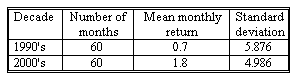### CFA Practice Question

There are 434 practice questions for this study session.

### CFA Practice Question

The realized mean monthly return on the S&P 500 in the 1990s appears to have been substantially different than the mean return in 2000s. The data indicate that assuming equal population variances is not unreasonable.μ1= 1990s population mean return and μ2 = 2000s population mean return.

The decision made in this hypothesis is (assume at the 10% level) ______.
A. to reject the null hypothesis
B. that the t-value is significant at the 0.1 level
C. not to reject the null hypothesis
Explanation: Pooled estimate of variance needs to be computed as follows:

S2p = [(60 - 1)(5.876)2 + (60 - 1)(4.986)2] / (60 + 60 - 2) = 29.694

Now determine the value of t. t = [(0.7 - 1.8) - 0] / [29.964/60 + 29.964/60]1/2 = -1.101

We reject null if t > 1.658 or t < -1.658 (t-value: t(118, 0.05) = 1.658). The t-value of -1.101 does not fit the rejection criteria. In other words we do not reject the null hypothesis and the t-value is not significant at the 0.1 level.